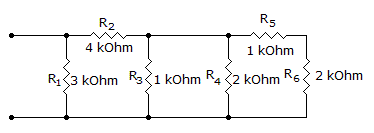# Electronics - Series-Parallel Circuits - Discussion

### Discussion :: Series-Parallel Circuits - General Questions (Q.No.28)

28.

What is the total resistance?[A]. 800[B]. 1.2 k[C]. 1.6 k[D]. 1.8 kExplanation:

No answer description available for this question.

 Prasanta said: (Jun 1, 2011) R=2k+1k+1/2k+1/1k+4k+1/3k =1.8 {d}

 Billy said: (Nov 12, 2014) Does anyone understand how to get the individual currents and voltages across each resistor. Can you please state the answer?

 Trixi said: (Mar 27, 2017) Here, R= 1 /(1/ R1 + 1/ R2 + 1/R3) + 1 / (1/ R3+ 1/ R4) + 1/ (1/ R4+ 1/ R5 + 1/R6).

 Sasi said: (Jul 17, 2017) How to solve if I have one more resistor in between R2 & R5?

 Shalu said: (May 26, 2018) Can anyone explain this in detail please?